Next: Form of solution from Up: Pattern from a single Previous: Meaning of infinitesimal

Relation between intensity and amplitude

Now, the intensity I (``brightness'' in the case of light) of the pattern at the observation screen is a just a measure of the amount of energy arriving at each point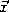on the screen per unit time. In a real experiment, the screen is a two-dimensional surface. Thus, the precise measure we use for the intensity is the average energy arriving per unit time per unit area, or, simply, average power per unit area. To relate this to the solution for the waves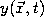, we can generalize from the result we know for power on a string,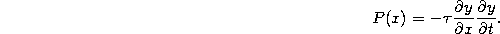It turns out that all phenomena (such as sound or light) which obey the wave-equation obey a very similar formula. The primary difference in going from waves on a string to any other type of wave is that the constant(which measures the tension of the string) is replaced by some other constant characterizing the medium of propagation for the waves. If we consider traveling sinusoidal waves, then the solution will have the general form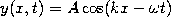. Then, using Eq. 1 we will find a result of the following form for the power. (Here,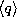indicates the average value of the quantity q.)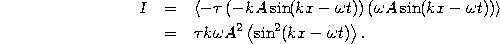Note that, at any instant in time t, the power may be different: it varies with the oscillations of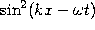. This is why we define intensity as the time average of the arriving power. Finally, because the time average of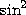or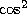is just 1/2, we have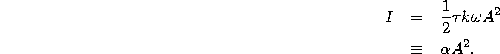Note that in the last line we replaced the factor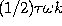with a general proportionality constant. We do this because for waves which are not waves on a string, we end up with factors other thanto describe the medium of propagation. What is the same for all types of waves, the main point here, is that the intensity is proportional to the square of the amplitude.Next: Form of solution from Up: Pattern from a single Previous: Meaning of infinitesimal

Tomas Arias
Thu Sep 13 15:26:14 EDT 2001